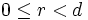# Difference between revisions of "Dihedral group"

WARNING: POTENTIAL TERMINOLOGICAL CONFUSION: Please don't confuse this with dicyclic group (also called binary dihedral group)
This article defines a group property: a property that can be evaluated to true/false for any given group, invariant under isomorphism
View a complete list of group properties
VIEW RELATED: Group property implications | Group property non-implications |Group metaproperty satisfactions | Group metaproperty dissatisfactions | Group property satisfactions | Group property dissatisfactions

This is a family of groups parametrized by the natural numbers, viz, for each natural number, there is a unique group (upto isomorphism) in the family corresponding to the natural number. The natural number is termed the parameter for the group family

## Definition

The dihedral group with parameter$n$, denoted sometimes as$D_n$ and sometimes as$D_{2n}$ is defined in the following equivalent ways:$\langle x,a|a^n = x^2 = e, xax^{-1} = a^{-1} \rangle$

• (For$n \ge 3$): It is the group of symmetries of a regular$n$-gon in the plane, viz., the plane isometries that preserves the set of points of the regular$n$-gon.

The dihedral groups arise as a special case of a family of groups called von Dyck groups.

Note that for$n = 1$ and$n = 2$, the geometric description of the dihedral group does not make sense. In these cases, we use the algebraic description.

## Elements

### In the case of twice an odd number

Suppose$n$ is odd and greater than$1$. Let$D_{2n}$ be the dihedral group of order$2n$. It has the following elements:

•$n$ elements of odd order, all of them in the cyclic subgroup$\langle a \rangle$ of order$n$. Of these, there are precisely$\varphi(d)$ elements of order$d$ for any divisor$d$ of$n$.
•$n$ elements of order$2$. These are the elements outside the cyclic subgroup$\langle a \rangle$.

The conjugacy classes of elements are as follows (a total of$(n+3)/2$ conjugacy classes):

• The identity element is its own conjugacy class.
• The non-identity elements in$\langle a \rangle$ occur in conjugacy classes of size two. Each element is conjugate in$D_{2n}$ to its inverse. Thus, these form$(n-1)/2$ conjugacy classes.
• The elements outside$\langle a \rangle$ all form a single conjugacy class of size$n$.

The equivalence classes of elements upto automorphism are as follows:

• Two elements inside$\langle a \rangle$ are related via an automorphism if and only if they generate the same cyclic subgroup.
• Any two elements outside$\langle a \rangle$ are related via an automorphism (in fact, they are in the same conjugacy class).

### In the case of twice an even number

Suppose$n = 2m$, and$D_{2n}$ is the dihedral group of order$2n$. Then,$D_{2n}$ has the following conjugacy classes (a total of$(n + 6)/2$ conjugacy classes):

• There are$(n+2)/2$ conjugacy classes inside$\langle a \rangle$: The identity element and$a^m$ are both central elements. All other elements have a conjugacy class of size two: the element and its inverse.
• There are two conjugacy classes outside$a$, of size$n/2$ each.

## Subgroups

There are two kinds of subgroups:

• Subgroups of the form$\langle a^d \rangle$, where$d|n$. The number of such subgroups equals the number of positive divisors of$n$, sometimes denoted$\tau(n)$. The subgroup generated by$a^d$ is a cyclic group of order$n/d$.
• Subgroups of the form$\langle a^d, a^r x \rangle$, where$d|n$ and$0 \le r < d$. The number of such subgroups equals the sum of all positive divisors of$n$, sometimes denoted$\sigma(n)$. The subgroup of the above form is a dihedral group of order$2n/d$.

In particular, all subgroups of the dihedral group are either cyclic or dihedral.

Also note that the dihedral group has subgroups of all orders dividing its order. This is true more generally for all finite supersolvable groups. Further information: Finite supersolvable implies subgroups of all orders dividing the group order

## Supergroups

### Groups having the dihedral group as quotient

The dicyclic group, also called the binary dihedral group, of order$4n$, has the dihedral group of order$2n$ as a quotient -- in fact the quotient by its center, which is a cyclic subgroup of order two. the presentation for the dicyclic group is given by:$\langle a,x \mid a^n = x^2 = (ax)^2 \}$.

Dicyclic groups whose order is a power of$2$ are termed generalized quaternion groups.

## Particular cases

### For small values

Note that all dihedral groups are metacyclic and hence supersolvable. A dihedral group is nilpotent if and only if it is of order$2^k$ for some$k$. It is abelian only if it has order$2$ or$4$.

Order of group Size of regular polygon it acts on Common name for the group Comment
4 2 Klein-four group elementary abelian group that is not cyclic
6 3 symmetric group:S3 metacyclic, hence supersolvable but not nilpotent
8 4 dihedral group:D8 nilpotent but not abelian
10 5 dihedral group:D10 metacyclic, hence supersolvable but not nilpotent